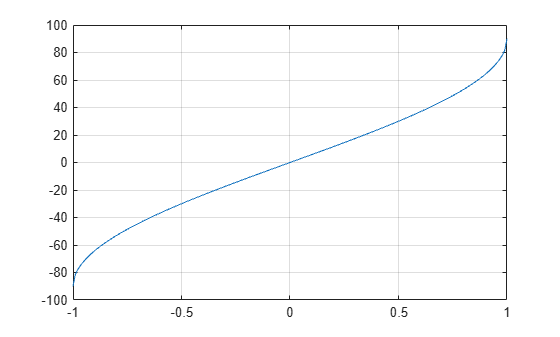# asind

Inverse sine in degrees

## Syntax

``Y = asind(X)``

## Description

example

````Y = asind(X)` returns the inverse sine (sin-1) of the elements of `X` in degrees. The function accepts both real and complex inputs. For real values of `X` in the interval [-1, 1], `asind(X)` returns values in the interval [-90, 90].For real values of `X` outside the interval [-1, 1] and for complex values of `X`, `asind(X)` returns complex values. ```

## Examples

collapse all

Show that the inverse sine of 1 is exactly 90°.

`asind(1)`
```ans = 90 ```

Show that the inverse sine, followed by sine, returns the original values of `X`.

`sind(asind([2 3]))`
```ans = 1×2 2 3 ```

Plot the inverse sine function over the domain $-1\le x\le 1$.

```x = -1:.01:1; plot(x,asind(x)) grid on```## Input Arguments

collapse all

Sine of angle, specified as a real-valued or complex-valued scalar, vector, matrix, or N-D array. The `asind` operation is element-wise when `X` is nonscalar.

Data Types: `single` | `double`
Complex Number Support: Yes

## Output Arguments

collapse all

Angle in degrees, returned as a real-valued or complex-valued scalar, vector, matrix, or N-D array of the same size as `X`.

## Version History

Introduced before R2006a